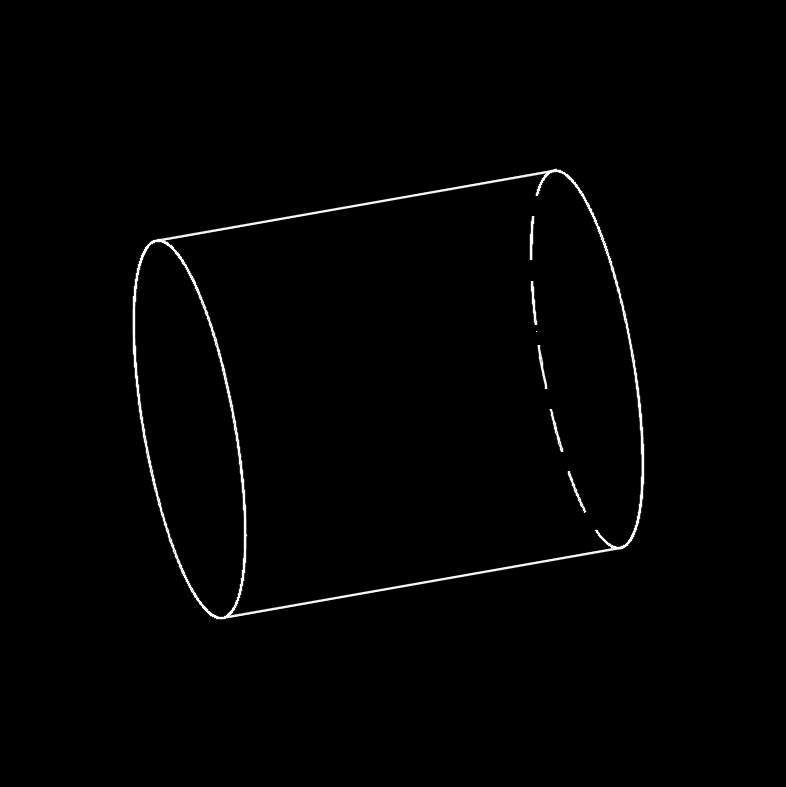# Radial Displacement Thin-wall Section (Internal Pressure Only)

on . Posted in Pressure Vessel## Radial Displacement Thin-wall Section (Internal Pressure only) Formula

$$\large{ \Delta_r = \frac{ p_i \; r_i^2 \; r }{ \lambda \; \left( r_e^2 \;-\; r_i^2 \right) } \; \left[ \left( 1 \;-\; \mu \right) + \left( 1 \;+\; \mu \right) \; \frac{ r_e^2 }{ r^2 } \right] }$$
Symbol English Metric
$$\large{ \Delta_r }$$ = radius change $$\large{ in }$$ $$\large{ mm }$$
$$\large{ \lambda }$$  (Greek symbol lambda) = elastic modulus $$\large{\frac{lbf}{in^2}}$$ $$\large{Pa}$$
$$\large{ \mu }$$  (Greek symbol mu) = Poisson's Ratio $$\large{ dimensionless }$$
$$\large{ p_i }$$ = internal pressure $$\large{\frac{lbf}{in^2}}$$ $$\large{Pa}$$
$$\large{ r }$$ = radius to point of intrest $$\large{ in }$$ $$\large{ mm }$$
$$\large{ r_e }$$ = external radius $$\large{ in }$$ $$\large{ mm }$$
$$\large{ r_i }$$ = internal radius $$\large{ in }$$ $$\large{ mm }$$